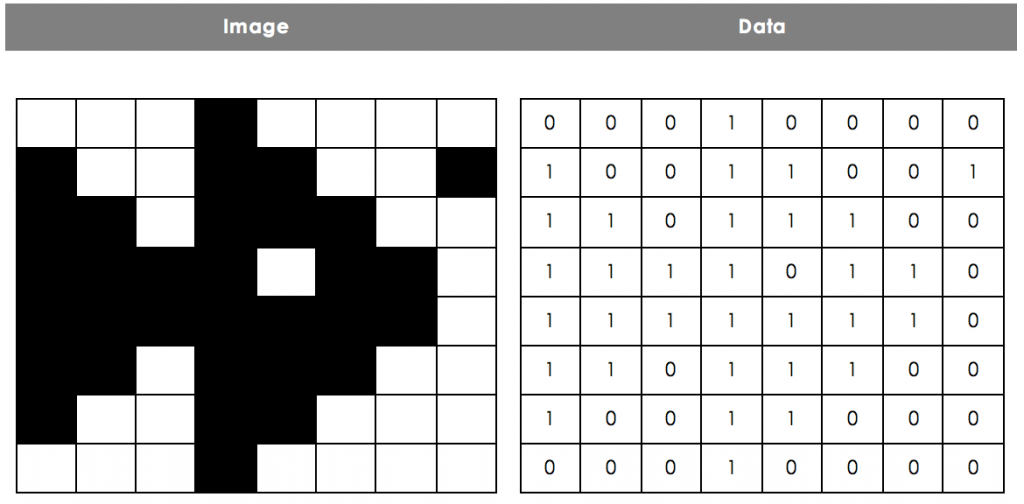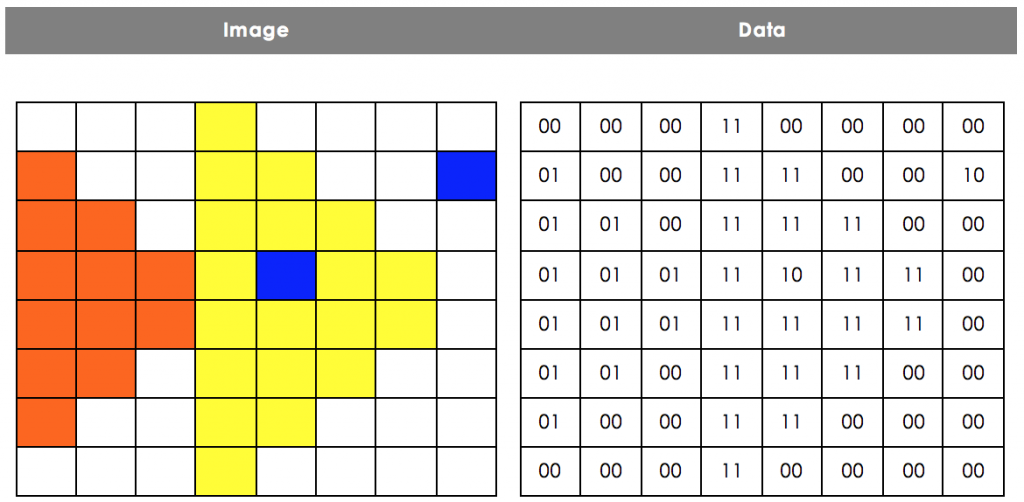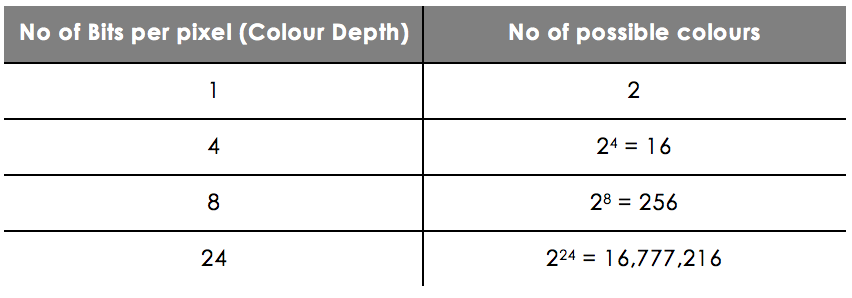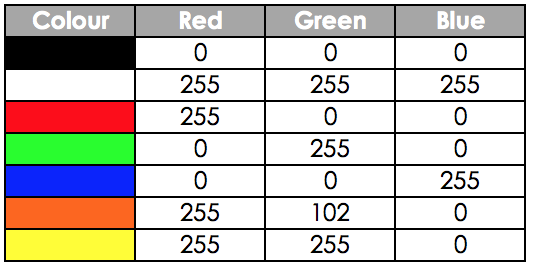# Image Encoding

## Bitmaps

Pixels

In order to store images on a computer, we need to have some way of encoding them using a binary representation. This means that all images stored on your computer are made up of a long string of 1s and 0s.One type of image stored on a computer is called a bitmap. All bitmap images are split into a grid of squares (picture elements), each called a pixel. The colour of each square is then stored as a single binary value.

This is most obvious in old video games where there was not enough memory and processing power to have complex images. For example, the heart on the left has 3 different colours (red, black and white), and it is clearly made up of several squares.

## Colour Depth

Different images can store different numbers of colours. A simple black and white image only needs to store two colours, black and white, and so an image could store the colours as a simple binary sequence.

The following binary sequence could be used to store this simple picture of a fish.

0001 0000 1001 1001 1101 1100 1111 0110 1111 1110 1101 1100 1001 1000 0001 0000In the example above a 1 represents black and a 0 represents white.

However, if we want to add more colours, we need to start increasing the number of bits used for each pixel. The number of bits used to store each colour is called the colour depth. Here is an example of an image stored using a colour depth of 2-bits.This time the binary representation would be twice as long.

0000 0011 0000 0000 0100 0011 1100 0010 0101 0011 1111 0000 0101 0111 1011 1100 0101 0111 1111 1100 0101 0011 1111 0000 0100 0011 1100 0000 0000 0011 0000 0000

In the image here, there are four different colours, each one represented by 2 binary digits. For example, 00 represents the colour white.

The colour depth determines how many colours can be represented on an image. With a colour depth of n bits, a computer can represent 2^n different colours. For example, if the colour depth is 1 bit, there are only 2^1 = 2 possible colours - black and white, whereas if is is 24 bits, there are 16,777,216 possibilities.What would be the binary representation of this image?
101001110
What number is used to represent Orange?
01
What number is used to represent Yellow?
11
What number is used to represent Blue?
10

## Image Resolution

The size of an image is measured by the number of pixels it is wide (width), by the number of pixels it is tall (height).

For example, an image described as 640 x 480 pixels would be 640 pixels wide and 480 pixels tall. Therefore, there would be a grid consisting of 480 rows of 640 pixels each - 307,200 pixels in total.

The number of pixels is referred to as the image resolution. The more pixels on the image, the clearer the image is, but also the bigger the file size.

For example, here are two files with the same image. The original had resolution of 2250 x 1499 and the other reduced to a resolution of 225 x 150. Both images have since been resized so that the difference in resolution can be clearly seen. For the original image files, the sizes were 646KB and 30KB respectively.

Resolution 2250 x 1500Low Resolution 225 x 150Image A: 480 x 640Image B: 800 x 600Which image has the higher resolution? Answer A or B.
B

## RGB Values

In general, photos use 3 bytes to store the colour of each pixel by using the number 0-255 __to represent the amount of each of Red, Green and Blue in the colour - the __RGB values. By mixing these three colours with different intensities, a computer display is able to show over 16 million different colours.

Here are some of the colours that can be made using different RGB values:## File Size

As mentioned before, the larger the file resolution (i.e. the more pixels there are) the bigger the file is - makes sense really! Also the greater the colour depth (i.e. the more bits we use to store each pixel) the bigger the file is.

If we know how many pixels we have (the width x height), and we know how many bits are used to store each pixel (the colour depth) we can use them to calculate the minimum file size of any image.

File size (bits) = width x height x colour depth

For example, a black and white image with colour depth of 1 and a resolution of 640 x 480 would require at least:

640 x 480 x 1 = 307200 bits = 38400 bytes = 38.4 kB

What is the minimum file size of this image (in bits)?
200
What is the minimum file size of this image (in bits)?
40
What is the minimum file size of this image (in bytes):
682
What is the minimum file size of this image (in KB)?
720
What is the minimum file size of this image (in KB)?
6912